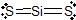# 3.11 Practice Problems

$$\newcommand{\vecs}{\overset { \rightharpoonup} {\mathbf{#1}} }$$ $$\newcommand{\vecd}{\overset{-\!-\!\rightharpoonup}{\vphantom{a}\smash {#1}}}$$$$\newcommand{\id}{\mathrm{id}}$$ $$\newcommand{\Span}{\mathrm{span}}$$ $$\newcommand{\kernel}{\mathrm{null}\,}$$ $$\newcommand{\range}{\mathrm{range}\,}$$ $$\newcommand{\RealPart}{\mathrm{Re}}$$ $$\newcommand{\ImaginaryPart}{\mathrm{Im}}$$ $$\newcommand{\Argument}{\mathrm{Arg}}$$ $$\newcommand{\norm}{\| #1 \|}$$ $$\newcommand{\inner}{\langle #1, #2 \rangle}$$ $$\newcommand{\Span}{\mathrm{span}}$$ $$\newcommand{\id}{\mathrm{id}}$$ $$\newcommand{\Span}{\mathrm{span}}$$ $$\newcommand{\kernel}{\mathrm{null}\,}$$ $$\newcommand{\range}{\mathrm{range}\,}$$ $$\newcommand{\RealPart}{\mathrm{Re}}$$ $$\newcommand{\ImaginaryPart}{\mathrm{Im}}$$ $$\newcommand{\Argument}{\mathrm{Arg}}$$ $$\newcommand{\norm}{\| #1 \|}$$ $$\newcommand{\inner}{\langle #1, #2 \rangle}$$ $$\newcommand{\Span}{\mathrm{span}}$$$$\newcommand{\AA}{\unicode[.8,0]{x212B}}$$

## Chemical Formulas

1. For the following molecules; write the chemical formula, determine how many atoms are present in one molecule/formula unit, determine the molar mass, determine the number of moles in 1.00 gram, and the number of grams in exactly 5.00 x 10-2 moles.

a) carbon dioxide

b) iron (II) chloride

c) dinitrogen pentoxide

d) iron (III) sulfate

Exercise $$\PageIndex{1}$$

 Formula Atoms per Molecule/Formula Unit Molar Mass Moles in 1.00 g Grams in 5.00 x 10-2 moles CO2 3 44.01 2.27 x 10-2 2.20 FeCl2 3 126.75 7.89 x 10-3 6.34 N2O5 7 108.02 9.26 x 10-3 5.40 Fe2(SO4)3 17 399.88 2.50 x 10-3 20.0

2. Name the following compounds, determine the molar mass, determine how many O atoms are present in one molecule/formula unit, determine the grams of oxygen in 1.00 mole of the compound, and determine how many moles of O atoms in 8.35 grams of the compound.

a) K2CO3

b) Ca(NO3)2

c) Cu2SO4

d) N2O

Exercise $$\PageIndex{2}$$

 Name Molar mass Atoms O per Molecule/Formula Unit Grams of O in 1.00 mole Moles of O in 8.35 grams potassium carbonate 138.205 3 48.0 1.81 x 10-1 calcium nitrate 164.088 6 96.0 3.05 x 10-1 copper(I) sulfate 223.25 4 64.0 1.50 x 10-1 dinitrogen monoxide 44.013 1 16.0 1.90 x 10-1

3. Give the chemical formula (including the charge!) for the following ions. Also, write out the chemical formula for the compound each anion would form with K+ and with Ca2+.

a) sulfate

b) sulfite

c) nitrate

d) chloride

e) nitride

f) acetate

g) carbonate

Exercise $$\PageIndex{3}$$

a) SO42-; K2SO4; CaSO4

b) SO32-; K2SO3; CaSO3

c) NO3-; KNO3; Ca(NO3)2

d) Cl-; KCl; CaCl2

e) N3-; K3N; Ca3N2

f) C2H3O2-; KC2H3O2; Ca(C2H3O2)2

g) CO32-; K2CO3; CaCO3

## Lewis Dot Structures

4. For the compounds i) SiS2, ii) PI3, iii) SCl2, and iv) CH2PH a) draw the Lewis dot structure, b) determine the hybridization of the central atom(s), c) determine the EGA around the central atom(s), d) determine the MG around the central atom(s), e) determine the bond angles in the molecule, f) determine if the molecule is polar or non-polar

Exercise $$\PageIndex{4}$$

i) a)b) sp c) line d) line e) 180o f) nonpolar

ii) a)b) sp3 c) tetrahedron d) trigonal pyramid e) <109.5o f) polar

iii) a)b) sp3 c) tetrahedron d) bent e) <109.5o f) polar

iv) a)b) C sp2 and N sp2 c) C and N trigonal plane d) C trigonal plane; N bent e) <120o f) polar

Modified by Tom Neils (Grand Rapids Community College)

3.11 Practice Problems is shared under a not declared license and was authored, remixed, and/or curated by LibreTexts.